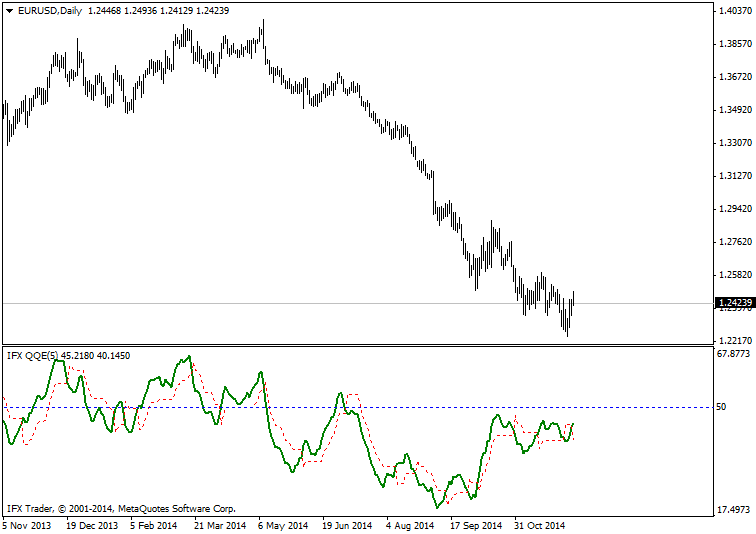#### Formula

Rsi_r:= (rsiprice – ref(rsiprice,-1));

Rsi_rs:= Wilders(if(rsi_r>0,rsi_r,0),rsiperiods) / Wilders(if(rsi_r<0,Abs(rsi_r),0),rsiperiods);

RSIndex:= Mov((100-(100/(1+rsi_rs))),SF,ST);

1. A buy signal is formed when the red line of IFX_QQE crosses the green line of the indicator upward. The upward crossing of 50 level by the green line is also considered as a buy signal.

2. A sell signal is created when the red line of IFX_QQE crosses the green line of the indicator downward. The downward crossing of 50 level by the green line also indicates a sell signal.#### IIFX_QQE parameters:

SmoothFactor - 5

﻿
अभी बात नहीं कर सकते?
अपना प्रश्न पूछें बातचीत.